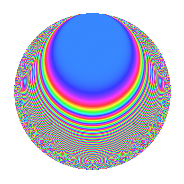# Properties

 Label 2005.2.a.eLevel 2005 Weight 2 Character orbit 2005.a Self dual Yes Analytic conductor 16.010 Analytic rank 1 Dimension 29 CM No

# Related objects

## Newspace parameters

 Level: $$N$$ = $$2005 = 5 \cdot 401$$ Weight: $$k$$ = $$2$$ Character orbit: $$[\chi]$$ = 2005.a (trivial)

## Newform invariants

 Self dual: Yes Analytic conductor: $$16.0100056053$$ Analytic rank: $$1$$ Dimension: $$29$$ Fricke sign: $$1$$ Sato-Tate group: $\mathrm{SU}(2)$

## $q$-expansion

The dimension is sufficiently large that we do not compute an algebraic $$q$$-expansion, but we have computed the trace expansion.

 $$\operatorname{Tr}(f)(q) =$$ $$29q - 5q^{2} - 3q^{3} + 19q^{4} - 29q^{5} - 6q^{6} + 12q^{7} - 15q^{8} + 14q^{9} + O(q^{10})$$ $$\operatorname{Tr}(f)(q) =$$ $$29q - 5q^{2} - 3q^{3} + 19q^{4} - 29q^{5} - 6q^{6} + 12q^{7} - 15q^{8} + 14q^{9} + 5q^{10} - 38q^{11} - 6q^{12} + 5q^{13} - 18q^{14} + 3q^{15} + 7q^{16} - 16q^{17} - 2q^{18} - 18q^{19} - 19q^{20} - 20q^{21} - 2q^{22} - 19q^{23} - 19q^{24} + 29q^{25} - 21q^{26} - 21q^{27} + 26q^{28} - 31q^{29} + 6q^{30} - 13q^{31} - 30q^{32} + 2q^{33} - 14q^{34} - 12q^{35} - 29q^{36} - q^{37} - 23q^{38} - 39q^{39} + 15q^{40} - 24q^{41} - 20q^{42} - 27q^{43} - 76q^{44} - 14q^{45} - 11q^{46} - 5q^{47} - 2q^{48} - 11q^{49} - 5q^{50} - 58q^{51} + 11q^{52} - 37q^{53} - 18q^{54} + 38q^{55} - 50q^{56} - 6q^{57} + 31q^{58} - 67q^{59} + 6q^{60} - 31q^{61} - 19q^{62} - 2q^{63} - 13q^{64} - 5q^{65} + 6q^{66} - 17q^{67} - 16q^{68} - 48q^{69} + 18q^{70} - 53q^{71} + 9q^{72} + 29q^{73} - 59q^{74} - 3q^{75} - 21q^{76} - 62q^{77} - 12q^{78} - 13q^{79} - 7q^{80} - 11q^{81} + 32q^{82} - 72q^{83} - 58q^{84} + 16q^{85} - 43q^{86} + 4q^{87} + 12q^{88} - 38q^{89} + 2q^{90} - 45q^{91} - 37q^{92} - 27q^{93} - 44q^{94} + 18q^{95} - 21q^{96} + 32q^{97} - 32q^{98} - 107q^{99} + O(q^{100})$$

## Embeddings

For each embedding $$\iota_m$$ of the coefficient field, the values $$\iota_m(a_n)$$ are shown below.

For more information on an embedded modular form you can click on its label.

Label $$a_{2}$$ $$a_{3}$$ $$a_{4}$$ $$a_{5}$$ $$a_{6}$$ $$a_{7}$$ $$a_{8}$$ $$a_{9}$$ $$a_{10}$$
1.1 −2.67547 2.10999 5.15811 −1.00000 −5.64522 2.87574 −8.44943 1.45208 2.67547
1.2 −2.65420 −1.14990 5.04475 −1.00000 3.05206 2.92882 −8.08137 −1.67773 2.65420
1.3 −2.47126 −1.08024 4.10715 −1.00000 2.66955 0.388284 −5.20731 −1.83308 2.47126
1.4 −2.27234 1.15962 3.16351 −1.00000 −2.63504 −2.94936 −2.64390 −1.65529 2.27234
1.5 −2.13554 1.65605 2.56051 −1.00000 −3.53655 1.78297 −1.19700 −0.257503 2.13554
1.6 −1.86230 −1.84094 1.46818 −1.00000 3.42839 3.02254 0.990420 0.389053 1.86230
1.7 −1.77645 −3.27205 1.15578 −1.00000 5.81263 2.00064 1.49972 7.70629 1.77645
1.8 −1.71603 −0.663123 0.944755 −1.00000 1.13794 −2.54726 1.81083 −2.56027 1.71603
1.9 −1.29166 0.182887 −0.331605 −1.00000 −0.236228 2.69438 3.01165 −2.96655 1.29166
1.10 −1.19798 2.37610 −0.564833 −1.00000 −2.84653 −3.68594 3.07263 2.64584 1.19798
1.11 −1.13376 2.41050 −0.714583 −1.00000 −2.73294 4.26135 3.07769 2.81053 1.13376
1.12 −0.706999 2.56202 −1.50015 −1.00000 −1.81135 −2.27480 2.47460 3.56397 0.706999
1.13 −0.524480 −2.05313 −1.72492 −1.00000 1.07683 −2.06215 1.95365 1.21534 0.524480
1.14 −0.475745 −1.88724 −1.77367 −1.00000 0.897847 −2.32576 1.79530 0.561689 0.475745
1.15 −0.370815 −1.61059 −1.86250 −1.00000 0.597230 4.02410 1.43227 −0.406010 0.370815
1.16 0.154915 0.533773 −1.97600 −1.00000 0.0826896 2.26312 −0.615943 −2.71509 −0.154915
1.17 0.282613 −0.0447289 −1.92013 −1.00000 −0.0126409 0.565532 −1.10788 −2.99800 −0.282613
1.18 0.562660 −3.02550 −1.68341 −1.00000 −1.70233 −2.33072 −2.07251 6.15366 −0.562660
1.19 0.609831 −1.81759 −1.62811 −1.00000 −1.10842 −0.599157 −2.21253 0.303632 −0.609831
1.20 0.631551 1.59060 −1.60114 −1.00000 1.00455 2.69469 −2.27430 −0.469979 −0.631551
See all 29 embeddings
 $$n$$: e.g. 2-40 or 990-1000 Embeddings: e.g. 1-3 or 1.29 Significant digits: Format: Complex embeddings Normalized embeddings Satake parameters Satake angles

## Inner twists

This newform does not have CM; other inner twists have not been computed.

## Atkin-Lehner signs

$$p$$ Sign
$$5$$ $$1$$
$$401$$ $$1$$

## Hecke kernels

This newform can be constructed as the intersection of the kernels of the following linear operators acting on $$S_{2}^{\mathrm{new}}(\Gamma_0(2005))$$:

 $$T_{2}^{29} + \cdots$$ $$T_{11}^{29} + \cdots$$